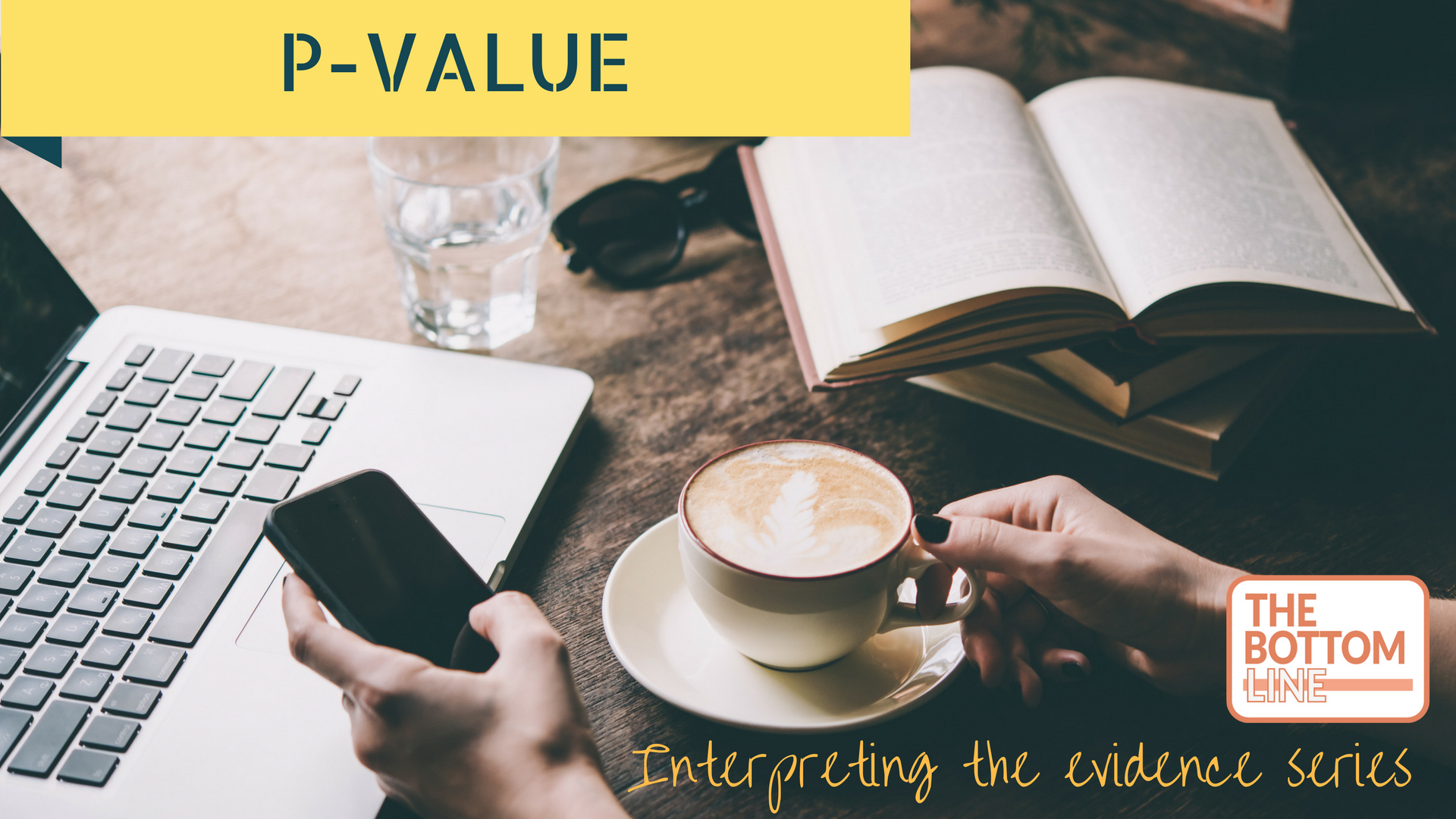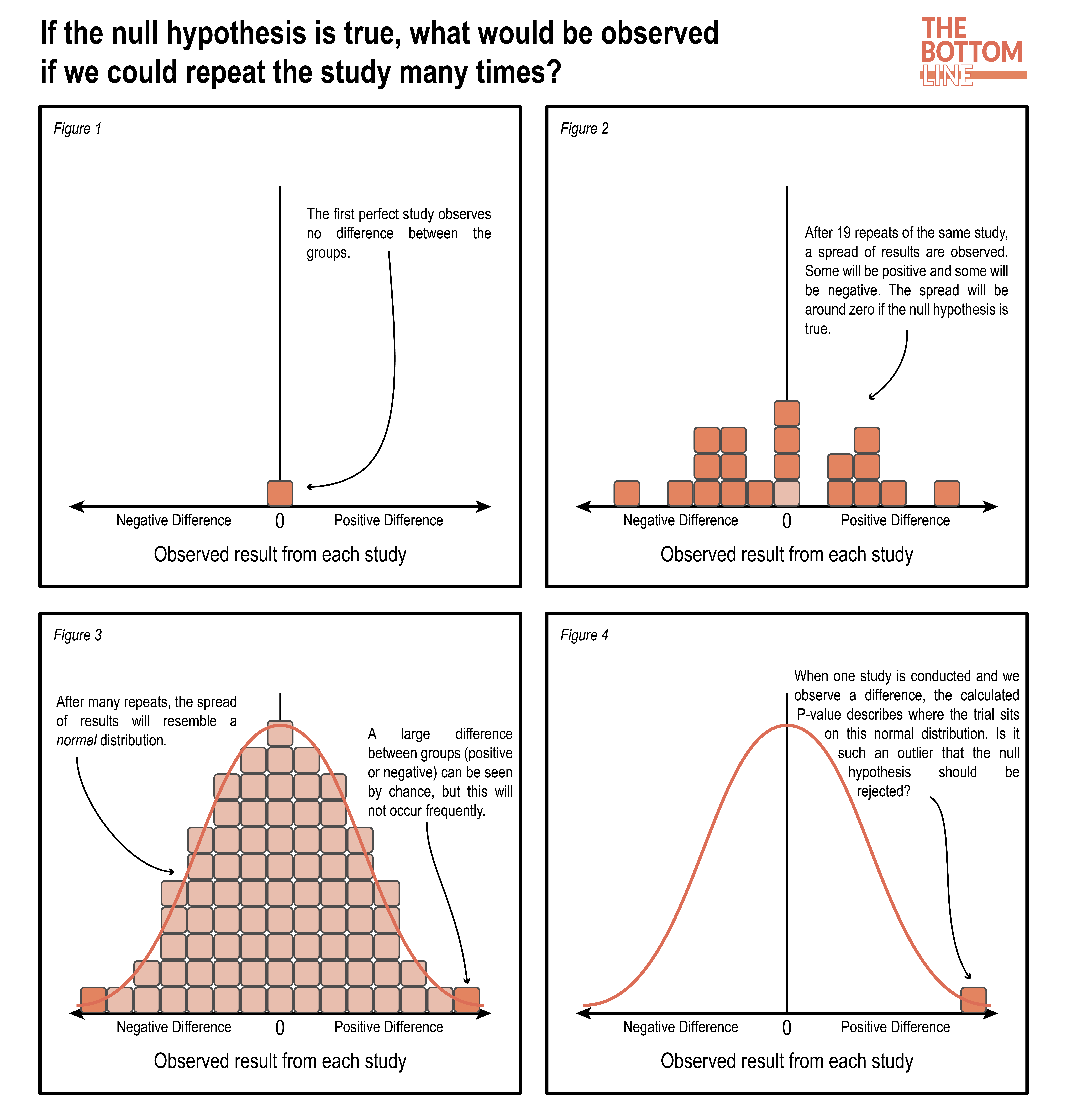# P-Value“The p-value is probably the most ubiquitous and at the same time, misunderstood, misinterpreted, and occasionally miscalculated index in all of biomedical research”

– Steven Goodman

### Definition of P-value

The probability of obtaining a result equal to, or “more extreme” than, that actually observed, under the assumption that the null hypothesis (there is no difference between specified populations) is correct.

### What does that mean?

Ronald Fisher (1890-1962), considered the father of modern statistical inference, introduced the idea of significance levels as a means of examining the discrepancy between the data and the null hypothesis.

“If P is between .1 and .9 there is certainly no reason to suspect the hypothesis tested. If it is below .02 it is strongly indicated that the hypothesis fails to account for the whole of the facts. We shall not often be astray if we draw a conventional line at .05”

– Ronald Fisher

Over time, the original meaning of the P-value has adapted, and this often results in confusion and over-emphasis of it’s importance. Researchers often wish to turn a P-value into a statement about the truth of a null hypothesis, or about the probability that random chance produced the observed data. The P-value is neither. It is a statement about data in relation to a specified hypothetical explanation, and is not a statement about the validity of the explanation itself.

A P-value of 0.05 infers, assuming the postulated null hypothesis is correct, any difference seen (or an even bigger “more extreme” difference) in the observed results would occur 1 in 20 (or 5%) of the times a study was repeated.

A P-value of 0.01 infers, assuming the postulated null hypothesis is correct, any difference seen (or an even bigger “more extreme” difference) in the observed results would occur 1 in 100 (or 1%) of the times a study was repeated.

The P-value tells you nothing more than this. Any further inference about whether study findings should be accepted or rejected should be based on evaluation of the study design, results of other statistical tests, the plausibility of the clinical question and outcomes, and evaluation of the strengths and limitations of a study.

###P-Pitfalls

• Much emphasis has been placed on the importance of the P-value, causing a danger that results are over interpreted. The P-value does not provide information about:
• Effect size – what is the clinical significance?
• A new drug for treating sepsis increases mean arterial blood pressure compared with placebo. If this difference in blood pressure is only 2mmHg, the effect size is small regardless of the statistical difference
• Power and sample size – For two identical studies: Study A includes 50 patients, whilst study B includes 5000 patients. Whilst both may have a P-value of 0.05, clearly study B, the larger study, is more likely to represent the wider population and have greater likelihood of demonstrating a true effect
• Confidence Intervals, risk reductions, NNT and Fragility Index
• A P-value is akin to a CRP in sepsis. It may indicate an important finding if the CRP is raised (or P-value is low), but more information is needed in order to have a more complete picture
• The P-value is not the probability that the test hypothesis is true
• A P-value of 0.05 does not mean there is a 5% chance of making a mistake
• A P-value of <0.05
• does not mean you have proved your experimental hypothesis
• does not mean that the result is clinically significant
• A P-value of > 0.05 does not mean there is no difference between groups
• P-values of 0.05 and ≤0.05 are not the same
• It is important to not just ignore study outcomes with a reported P-value > 0.05. A well conducted study with important clinical outcomes but a P-value of 0.51 should arguably demand more attention than an inferior study with a P-value of 0.05

The ASA statement on P-values is available here

### The Bottom Line

• A P-value indicates the degree to which the data conform to the pattern predicted by the experimental hypothesis and all the other assumptions used in the test (the underlying statistical model)
• P-values are based on the null hypothesis being correct, and that any measured difference between groups may be due to chance if the value is very low. We might infer that the alternative hypothesis is correct if the P-value is very low, but importantly it does not actually test for this
• Correct and careful interpretation of statistical tests demands examining the sizes of effect estimates, confidence intervals, as well as precise P-values
• The Fragility Index of RCTs may help readers make more informed decisions about the confidence warranted by RCT results. It is recommended that this is used alongside the P-value and other suitable analyses

Summary author: Steve Mathieu
Summary date: 14th April 2017
Peer-review editor: Charlotte Summers

### One comment

•J Verden

Hello
I would like to point out that although this article is seeking to reconcile the correct understanding of a p-value, with two statements it is actually perpetuating misunderstanding:

Quote:
A P-value of 0.05 infers, assuming the postulated null hypothesis is correct, any difference seen (or an even bigger “more extreme” difference) in the observed results would occur 1 in 20 (or 5%) of the times a study was repeated.

A P-value of 0.01 infers, assuming the postulated null hypothesis is correct, any difference seen (or an even bigger “more extreme” difference) in the observed results would occur 1 in 100 (or 1%) of the times a study was repeated.

According to the literature these two statements again perpetuate common misuses of the p-value:
1. the tendency to equate the decimal values originally cited by Fisher with percentages.
2. that it makes comment on what might occur with repeated testing – this is the domain of the other pioneers of scientific method Neyman and Pearson who did not use p-values.

Fishers p-value (null hypothesis significance testing) makes no comment on either percentage or arriving at these same results if the test was repeated.
Two investigators could seek to answer the same question, acquire their data and their data will generate different p-values and all that can be said is if it is below the classic 0.05 then the greater the unlikelihood that this data showing a difference could occur if the null hypothesis were true. That is all.

Ref:
Tam et al (2018) How doctors conceptualise P values: A mixed methods study AGP 47(10)
Gao (2020) P-values – a chronic conundrum BMC Medical Research Methodology 167

This site uses Akismet to reduce spam. Learn how your comment data is processed.